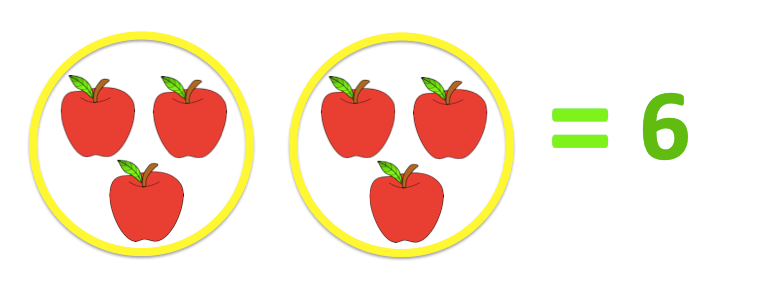# What is multiplication?

Multiplication is a mathematical process of repeated addition of the same number. The symbol for multiplication is ‘x’.

What is two times three? This just means two lots of three. The answer is six.For example:

2 x 4 = 8

5 x 3 = 15

3 x 2 = 5

8 x 8 = 64

4 x 5 = 20

6 x 3 = 18

9 x 3 = 27

# What is division?

Division is the opposite of multiplication. It is a mathematical process of breaking numbers into equal parts. The symbol for subtraction is ‘÷’.

For basic subtraction, you can use your finger to count. For larger numbers, you can use beads or items to help you subtract.

What is four divided by two? In order to work this out, we need to find how many equal parts can be made. The answer is that two equal parts can be made.

For example:

6 ÷ 2 = 3

8 ÷ 4 = 2

10 ÷ 5 = 2

12 ÷ 3 = 4

15 ÷ 5 = 3

# What is multiplication?

Multiplication is a mathematical process of repeated addition of the same number. The symbol for multiplication is ‘x’.

What is two times three? This just means two lots of three. The answer is six.

🍎🍎 x 🍎🍎🍎 = 🍎🍎🍎🍎🍎🍎

2      x       3       =          6

For example:

2 x 4 = 8

5 x 3 = 15

3 x 2 = 5

8 x 8 = 64

4 x 5 = 20

6 x 3 = 18

9 x 3 = 27

# What is division?

Division is the opposite of multiplication. It is a mathematical process of breaking numbers into equal parts. The symbol for subtraction is ‘÷’.

For basic subtraction, you can use your finger to count. For larger numbers, you can use beads or items to help you subtract.

What is four divided by two? In order to work this out, we need to find how many equal parts can be made. The answer is that two equal parts can be made.

🍎🍎🍎🍎 ÷ 🍎🍎 = 🍎🍎

4         ÷      2    =     2

For example:

6 ÷ 2 = 3

8 ÷ 4 = 2

10 ÷ 5 = 2

12 ÷ 3 = 4

15 ÷ 5 = 3